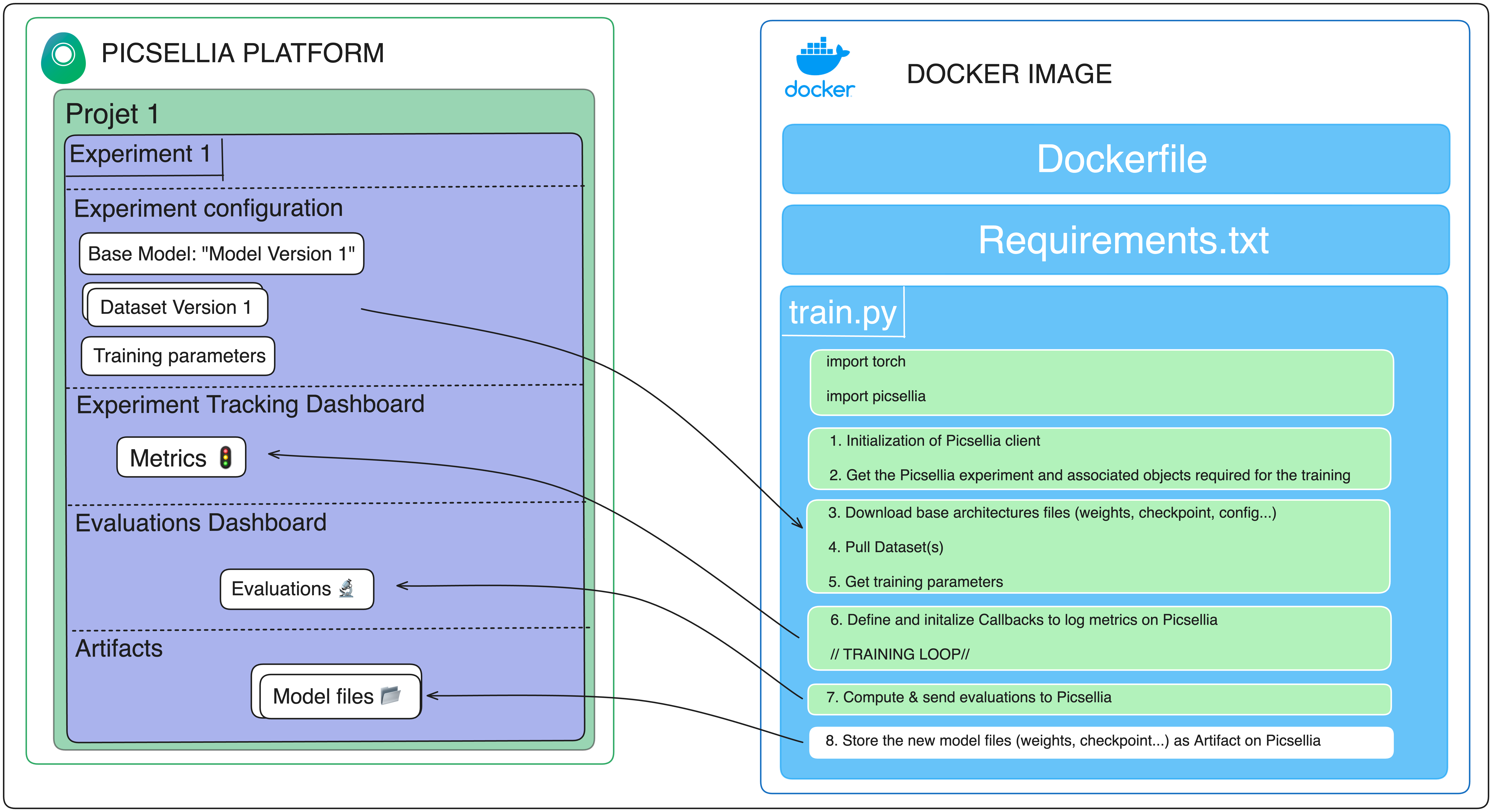# 5. Test your ModelVersion with Picsellia Evaluation Interface

At this point, the training of our `ModelVersion` should be completed. It is now time to use the Picsellia Evaluation Interface to determine how your newly trained `ModelVersion` is performing on a dedicated test set.

To accomplish this, we need to compute `Evaluation` and record them to the Picsellia Evaluation Interface at the end of our training script.

``````# ...

prediction = model.predict(ds_test) # Performing test on your testing dataset
picsellia_ds_test = experiment.get_dataset('test') # fetching picsellia test dataset
labels = list(ds_test.class_indices.keys()) # finding the class_names
labels_picsellia = {k: picsellia_ds_test.get_label(k) for k in labels} # building the picsellia label mapping

for i, pred in enumerate(prediction):
fname = ds_test.filenames[i].split('/')[-1]
asset = picsellia_ds_test.find_asset(filename=fname)
conf_score = float(np.max(pred))
class_name = labels[np.argmax(pred)]
picsellia_label = labels_picsellia[class_name]
``````

Let's take a look at each step:

1. To perform testing, get the `DatasetVersion` you wish to use. In this case, the `DatasetVersion` will be referred to as test.
``````test_dataset = experiment.get_dataset('test')
``````
1. Get the `Asset` you want to attach the test result to:
``````asset = test_dataset.find_asset(filename="filename.png")
``````
1. Initialize an object with your Picsellia `Label` in order to reduce the amount of API calls to perform 🌱
``````labels_picsellia = { k: picsellia_ds_test.get_label(k) for k in labels }
``````
1. Retrieve the results of your `ModelVersion` (which can be a `CLASSIFICATION`, `OBJECT_DETECTION`, or `SEGMENTATION`) and match it with your Picsellia `Label`. Using the example of `CLASSIFICATION`
``````conf_score = float(np.max(pred))
class_name = label_names_list[np.argmax(pred)]
picsellia_label = labels_picsellia[class_name]
``````
1. Add the test results to Picsellia Evaluation Interface
``````experiment.add_evaluation(asset, classifications=[(picsellia_label, conf_score)])
``````
1. Compute evaluation metrics
``````job = experiment.compute_evaluations_metrics(InferenceType.CLASSIFICATION)
job.wait_for_done()
``````